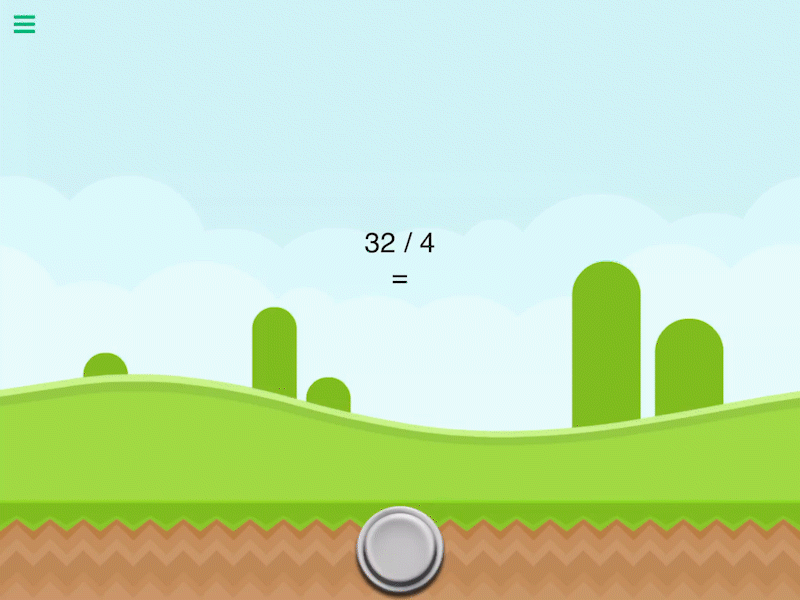# Divide Values

Description

Divide a number by another number. This will output the quotient of the 2 numbers entered.

Outputs

 Divide Value Outputs the quotient of two inputs.

Examples

10 divided by 2 outputs 5.

Related

Modulus

Multiply Values

Square Root

Subtract Values